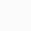# Know About XXV and CXXV Roman Numerals in Detail

Share With Friends or Family

What's In This Post?

## Know About XXV and CXXV Roman Numerals in Detail

As the name says, Roman numerals originated in ancient Rome. It was popular not only in Rome but throughout Europe. It swiftly spread throughout Europe, but its popularity plummeted after the Roman Empire fell out of power. Roman numerals, on the other hand, are still employed in a variety of applications today.

Let’s learn about two commonly used roman numerals “XXV and CXXV Roman Numerals” in detail with their definition, conversion into numbers and examples.

#### XXV Roman Numerals

The Roman numeral for 25 is XXV. The Roman numeral is an additive and subtractive system that uses letters to represent numbers in the number system. The Roman numerals that incorporate the English alphabet are represented by a distinct set of symbols. As a result, the number 25 can be written as XXV in roman numerals.

#### XXV Roman Numerals Conversion into Numbers

Roman numerals are not represented by digits, but rather by alphabets such as X, XI… and so on. Some alphabets, such as ‘M,’ stand for a large number, such as ‘thousand.’ Let’s examine how to convert them to numbers by using the steps below:

• To begin, we must understand that roman numerals are a combination of one or more alphabets, thus we must add the value of one alphabet to the value reflected by the alphabet/alphabets. For example, ‘I’ stands for 1 (one), IV stands for 4 (four), and X stands for 10 (Ten).

• Identify the alphabets, their values, and the order in which they are put. For example, in XXV Roman Numerals, X symbolises ten (10), followed by X again, implying ten (10) and finally V, implying five (5), therefore we will sum all the numbers, which in this case is 10 + 10 + 5 = 25. Because all of the alphabets are added together to reach the target number, it is also known as the Addition Method. When a larger alphabet follows a smaller alphabet, they are combined to produce the desired result.

• Subtracting the alphabets that are placed before and after it is another method that is occasionally utilised. For instance, the letter IV stands for “four,” whereas the letters I and V stand for “one” and “five,” respectively. To find the answer, one subtracts the letter after the next one, so “IV” is gotten by subtracting “I” from “V,” or “5 – 1 = 4.” This is also known as the subtraction method. It should be noted that to get the required outcome, the larger alphabet is subtracted if a smaller letter comes after it.

Another thing to remember is that you can only write or add the same number three (3) times in a row, for instance, XXX is 10+10+10=30. But XXXX is invalid because it doesn’t equal 40.

#### CXXV Roman Numerals

By combining the altered roman numerals, CXXV = C + X + X + V = 100 + 10 + 10 + 5 = 125, one can write the CXXV roman numerals as integers. The accurate translation of CXXV Roman Numerals results from the higher roman numerals coming before the lower ones.

#### How to Write CXXV Roman Numerals?

Any of the two methods shown below can be used to determine the numerical value of Roman numerals CXXV:

• With this method, we separate the roman numerals into individual letters, write the letter’s numerical value, and add or subtract the letters.

CXXV = C + X + X + V = 100 + 10 + 10 + 5 = 125

• In this method, the groupings of roman numerals for addition or subtraction are taken into consideration, such as,

CXXV

⇒ C + XX + V

⇒100 + 20 + 5

⇒125

CXXV roman numerals hence have a numerical value of 125.